# Lions Weekly: December 8, 2021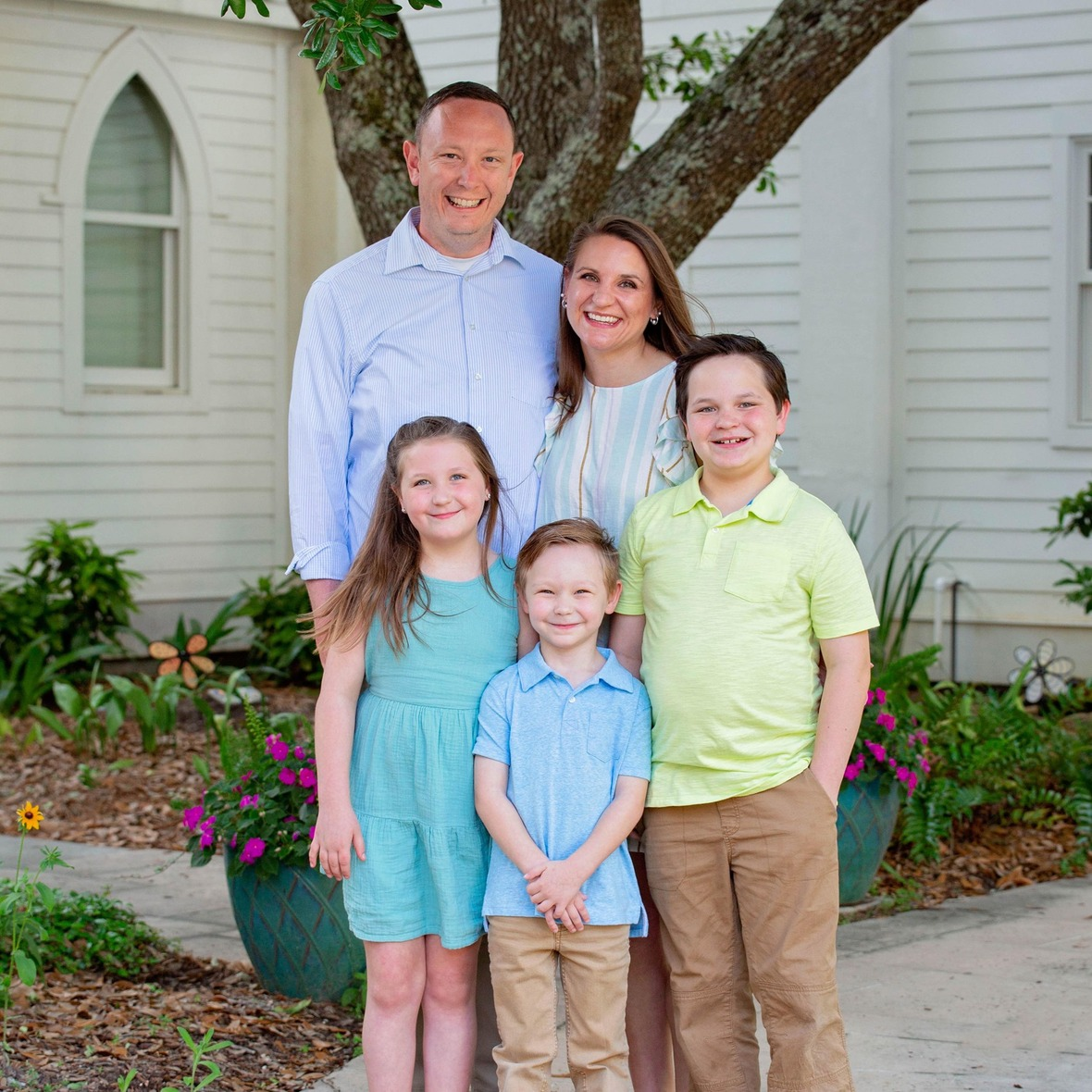# A Note From Mr. Sandefur

Lion Family,

Good afternoon! This past week we had the opportunity to usher in the Christmas season with productions from our band and choral music departments. Those in attendance were truly blessed as we celebrated the true reason for the season, the birth and life of Jesus Christ. I want to thank all of our students and staff who participated as they did an incredible job of faithfully using their gifts and talents to glorify God. There are two more opportunities this week to celebrate the meaning of Christmas as our pre-K and elementary students hold their program Thursday evening, and on Saturday we will host a special screening of “The Chosen” Christmas program. If you are able, please plan on joining us as we celebrate the true meaning of Christmas.

While we are actively celebrating Christmas, we are also working to finish the academic semester as strongly as possible. Please be sure to check your student’s grades and communicate with teachers as the semester draws to an end. Let us strive together to finish with excellence.

In Christ Alone,

Jeremy Sandefur
President

“The true light, which gives light to everyone, was coming into the world. He was in the world, and the world was made through him…” – John 1:9-10

 table div table+table+table+table div table{width:100%;padding:0}table div table+table+table+table div table img{width:96.23%;padding:0;float:none}table div table+table+table+table div table td{width:100%;padding:0 1.88% 18px}/* styles */# Save the Upcoming Dates!

As the Christmas season is approaching, we want to invite you to attend the many different Christmas celebrations happening on campus! Save the dates for the upcoming events listed below and celebrate the Christmas season with us at TKA!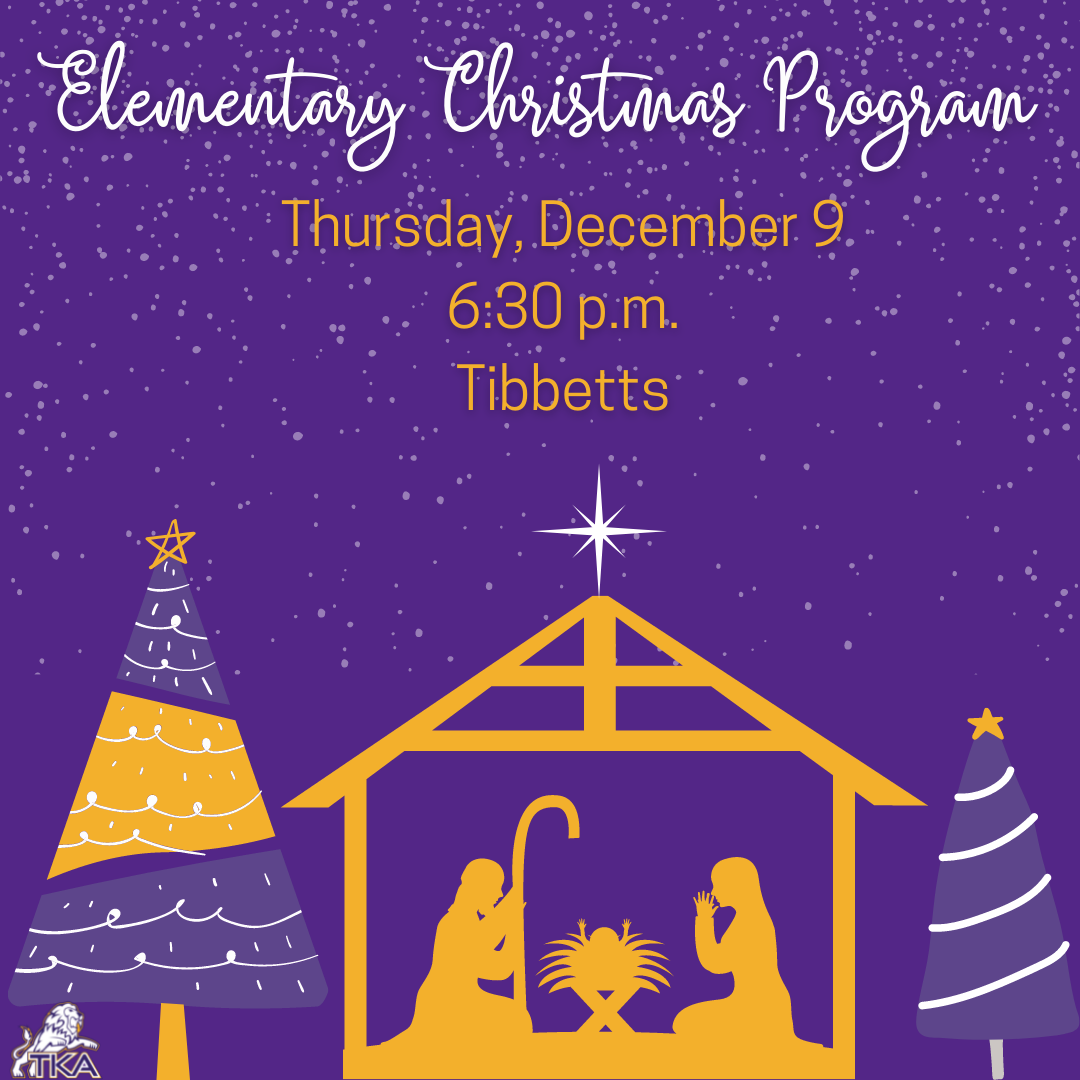# Elementary Christmas Program

Our elementary Christmas program will take place on Thursday, December 9, in Tibbetts. We are looking forward to celebrating the Christmas season with our loved ones through this program.

Scan the link below to view the program for this performance.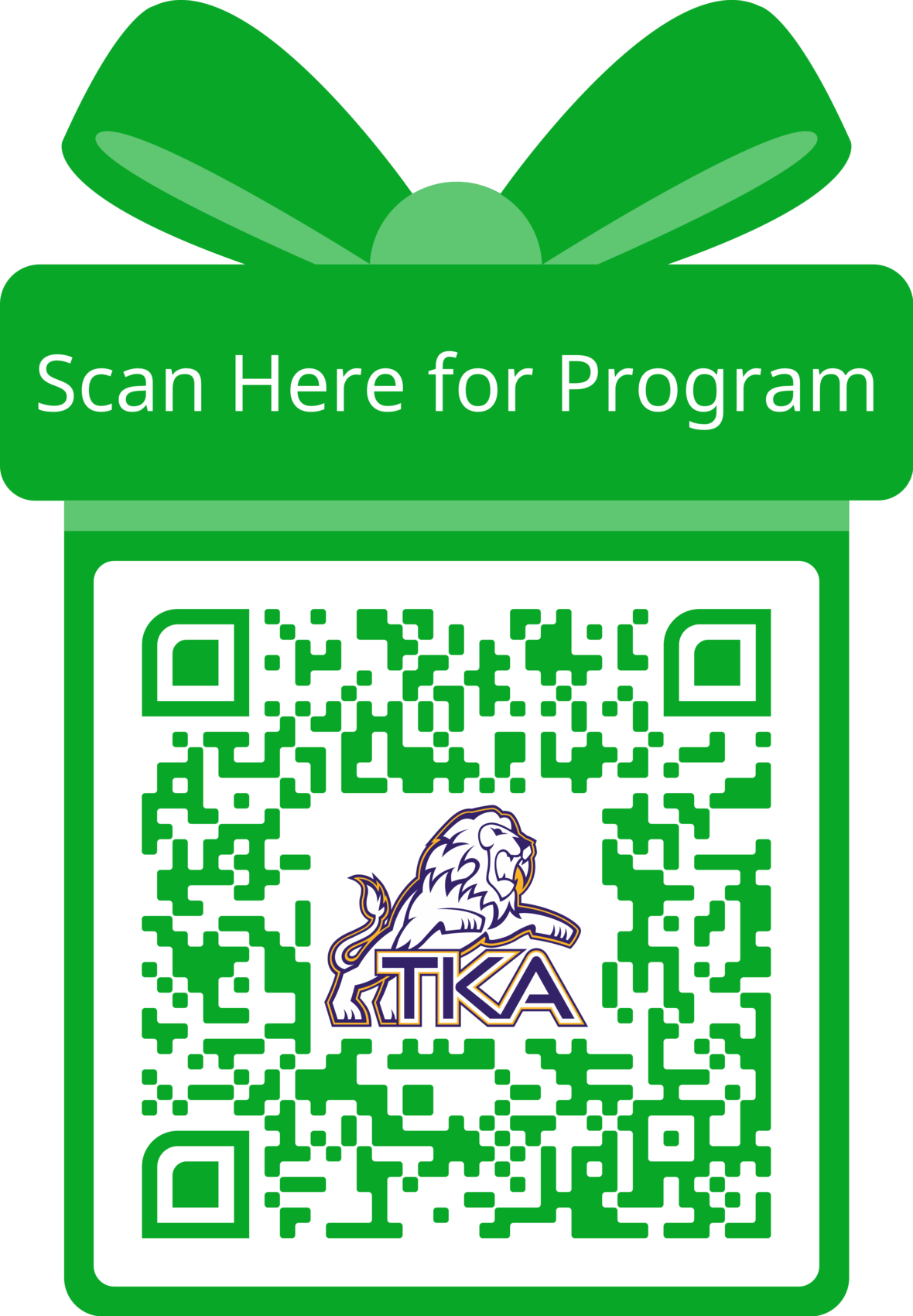table div table+table+table+table+table+table+table+table div table{width:100%;padding:0}table div table+table+table+table+table+table+table+table div table img{width:96.23%;padding:0;float:none}table div table+table+table+table+table+table+table+table div table td{width:100%;padding:0 1.88% 18px}/* styles */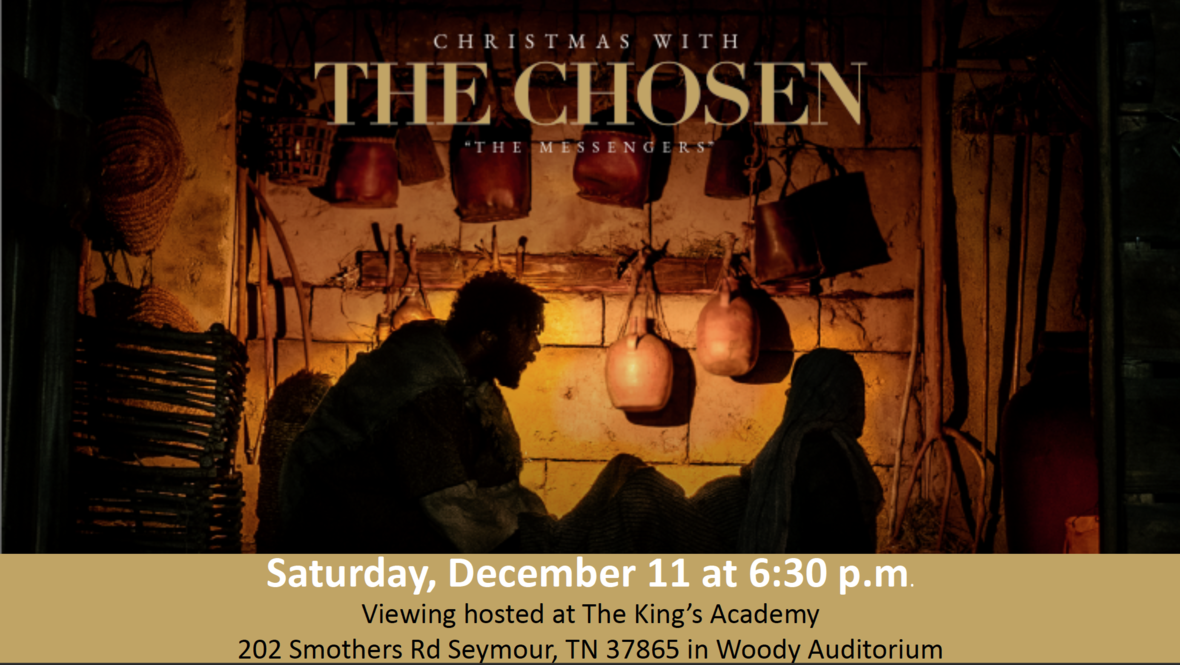Click the image to view The Chosen trailer

# Movie Showing

The King's Academy has been selected to host a viewing of "Christmas with The Chosen: The Messengers". To launch the Christmas season this December, The Chosen has created a special episode about the birth of Christ through the eyes of Mary and Joseph. You’ll also see an extraordinary lineup of musicians perform both new and classic Christmas songs from the set of The Chosen, including Phil Wickham, For King & Country, Brandon Lake, Maverick City Music, Cain, Matt Maher, Bryan & Katie Torwalt, Jordan Feliz, We The Kingdom, The Bonner Family, Leanna Crawford, Dawson Hollow, and One Voice Choir.

This is the perfect opportunity to bring friends and family, including those who haven’t seen The Chosen, for a unique Christmas experience.

Join us on Saturday, December 11 at 6:30 p.m. in Woody auditorium for this special event! Tickets for this event can be purchased by clicking here.

 table div table+table+table+table+table+table+table+table+table+table div table{width:100%;padding:0}table div table+table+table+table+table+table+table+table+table+table div table img{width:96.23%;padding:0;float:none}table div table+table+table+table+table+table+table+table+table+table div table td{width:100%;padding:0 1.88% 18px}/* styles */# Dr. Carroll Reece Reagan Pavilion Dedication Ceremony

Join us Friday, December 17, at 10:00 a.m. for the dedication ceremony for the Dr. Carroll Reece Reagan Pavilion.

 table div table+table+table+table+table+table+table+table+table+table+table+table div table{width:100%;padding:0}table div table+table+table+table+table+table+table+table+table+table+table+table div table img{width:96.23%;padding:0;float:none}table div table+table+table+table+table+table+table+table+table+table+table+table div table td{width:100%;padding:0 1.88% 18px}/* styles */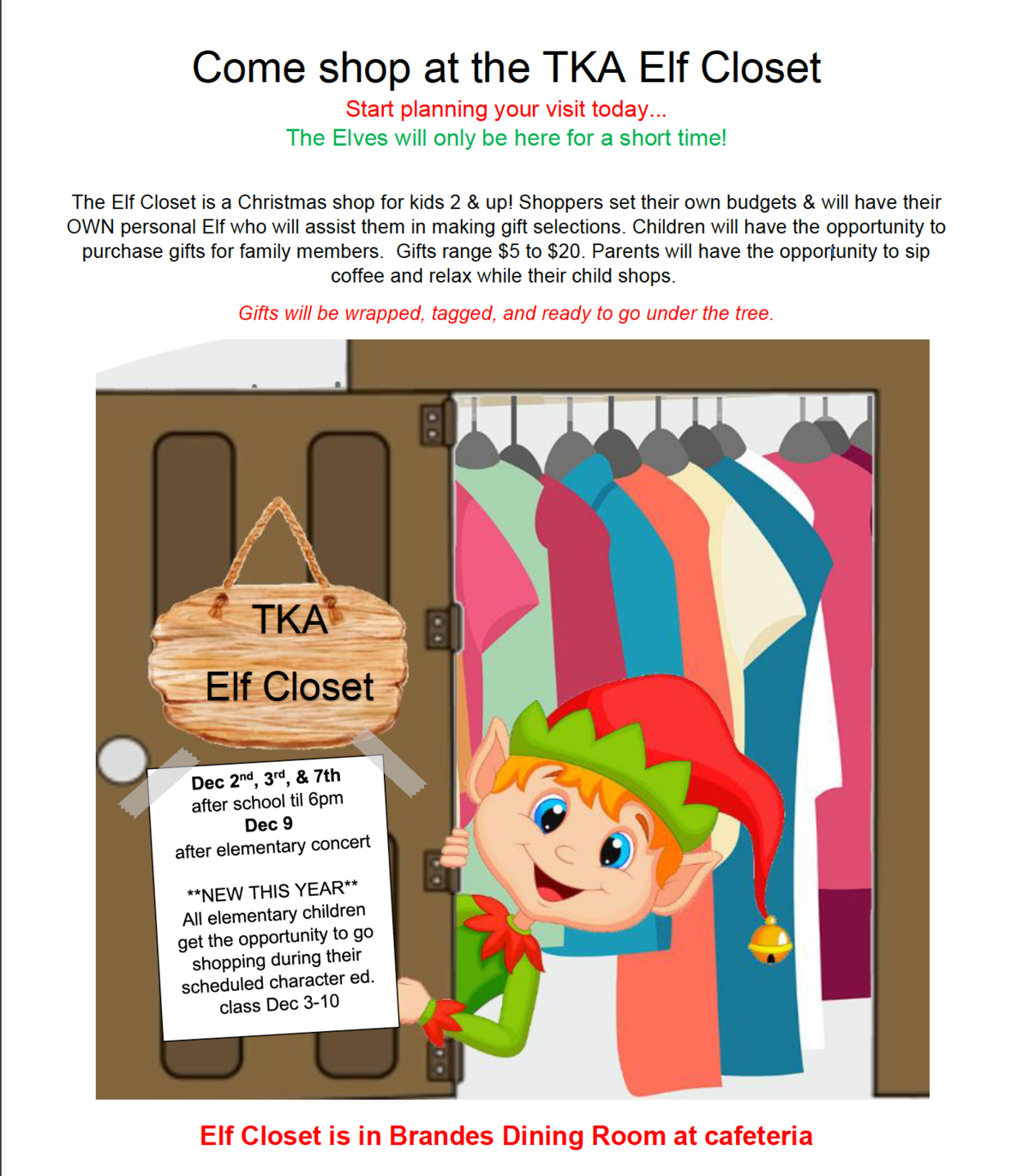# Elf Closet

Come shop at the TKA Elf Coset! The Elves will only be here for a short time.

The Elf Closet is a Christmas shop for kids 2 & up! Shoppers set their own budgets & will have their OWN personal Elf who will assist them in making gift selections. Children will have the opportunity to purchase gifts for family members. Gifts range \$5 to \$20. Parents will have the opportunity to sip coffee and relax while their child shops. Gifts will be wrapped, tagged, and ready to go under the tree.

For questions about the Elf Closet or to volunteer to help, contact Sarah Knight at 865-621-0741.

 table div table+table+table+table+table+table+table+table+table+table+table+table+table+table div table{width:100%;padding:0}table div table+table+table+table+table+table+table+table+table+table+table+table+table+table div table img{width:96.23%;padding:0;float:none}table div table+table+table+table+table+table+table+table+table+table+table+table+table+table div table td{width:100%;padding:0 1.88% 18px}/* styles */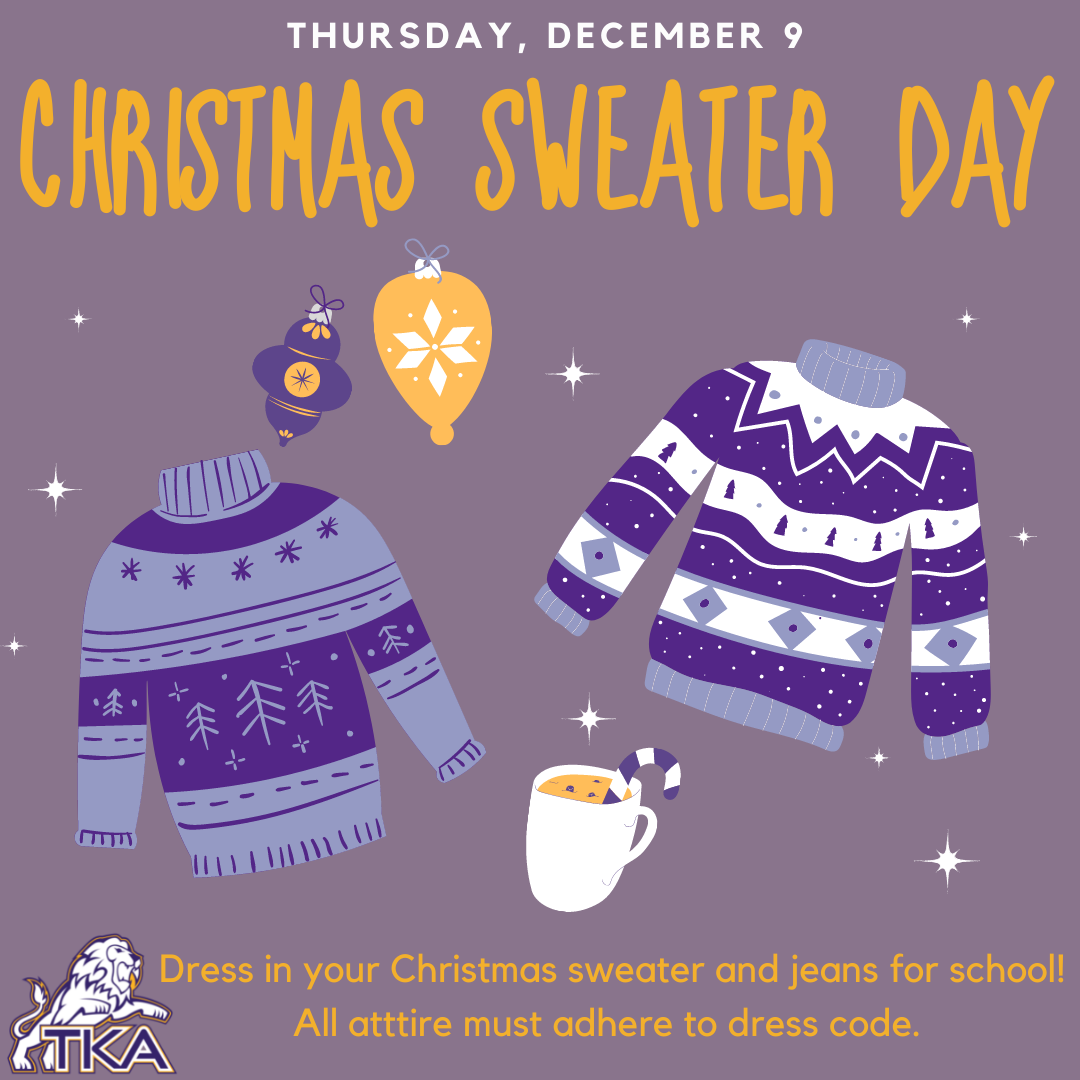# Christmas Sweater Day

On Thursday, December 9, students can dress in their favorite Christmas Sweater and jeans for school! There is no cost to participate in this day.

Remember, all attire must adhere to dress code.

 table div table+table+table+table+table+table+table+table+table+table+table+table+table+table+table+table div table{width:100%;padding:0}table div table+table+table+table+table+table+table+table+table+table+table+table+table+table+table+table div table img{width:96.23%;padding:0;float:none}table div table+table+table+table+table+table+table+table+table+table+table+table+table+table+table+table div table td{width:100%;padding:0 1.88% 18px}/* styles */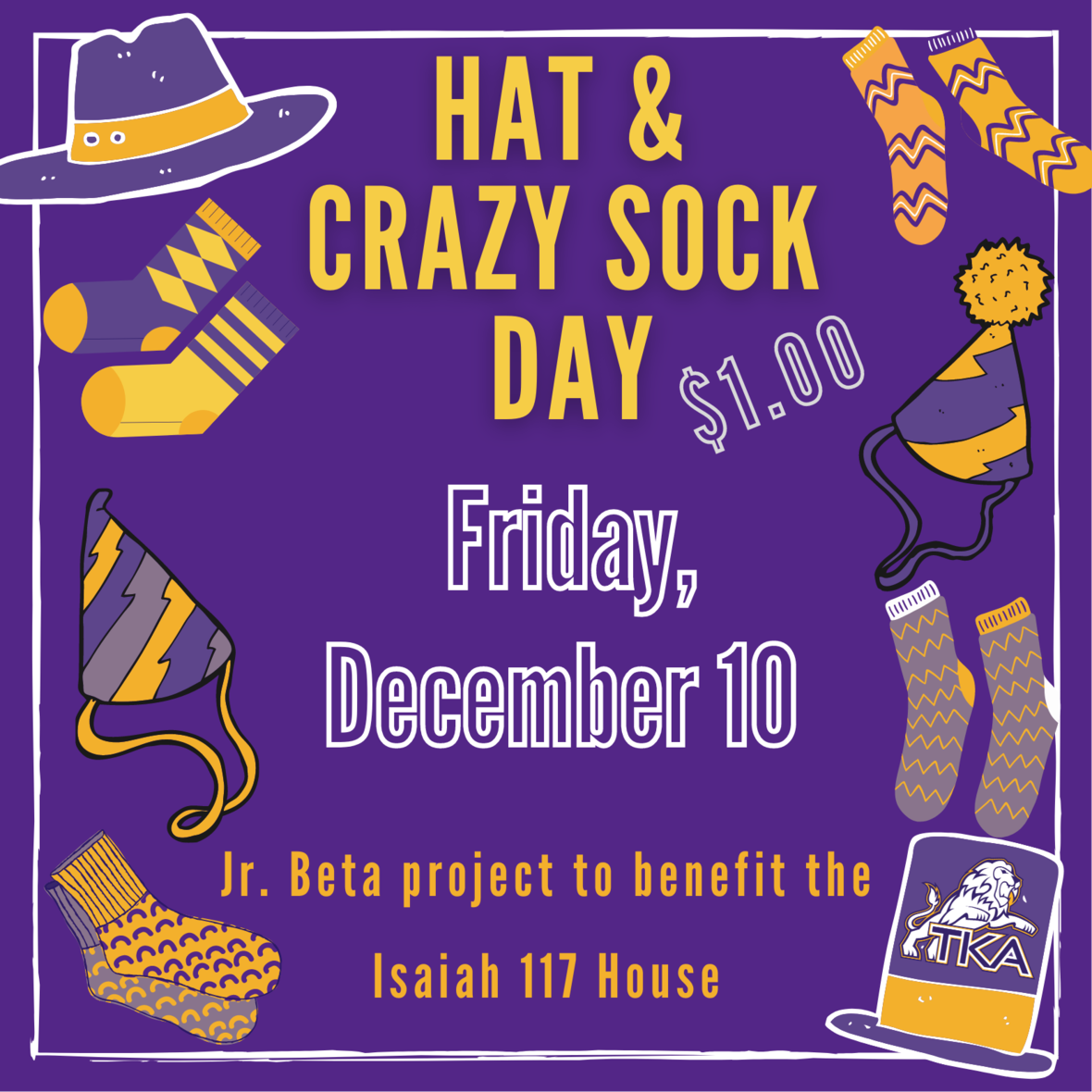# Hat and Crazy Sock Day

Jr. Beta is hosting a Hat and Crazy Sock Day to benefit the Isaiah 117 House.

Students can bring a \$1.00 (or more donation) to participate, OR students can bring a new toboggan to donate to the Isaiah 117 House. All financial and toboggan donations will be given directly to the Isaiah 117 House.

 table div table+table+table+table+table+table+table+table+table+table+table+table+table+table+table+table+table+table div table{width:100%;padding:0}table div table+table+table+table+table+table+table+table+table+table+table+table+table+table+table+table+table+table div table img{width:96.23%;padding:0;float:none}table div table+table+table+table+table+table+table+table+table+table+table+table+table+table+table+table+table+table div table td{width:100%;padding:0 1.88% 18px}/* styles */# Elementary Clubs

All fall elementary clubs will end this week by Friday, December 10.

For those who would like to participate in elementary clubs for the spring semester, sign up forms and information will be shared in January.

 table div table+table+table+table+table+table+table+table+table+table+table+table+table+table+table+table+table+table+table+table div table{width:100%;padding:0}table div table+table+table+table+table+table+table+table+table+table+table+table+table+table+table+table+table+table+table+table div table img{width:96.23%;padding:0;float:none}table div table+table+table+table+table+table+table+table+table+table+table+table+table+table+table+table+table+table+table+table div table td{width:100%;padding:0 1.88% 18px}/* styles */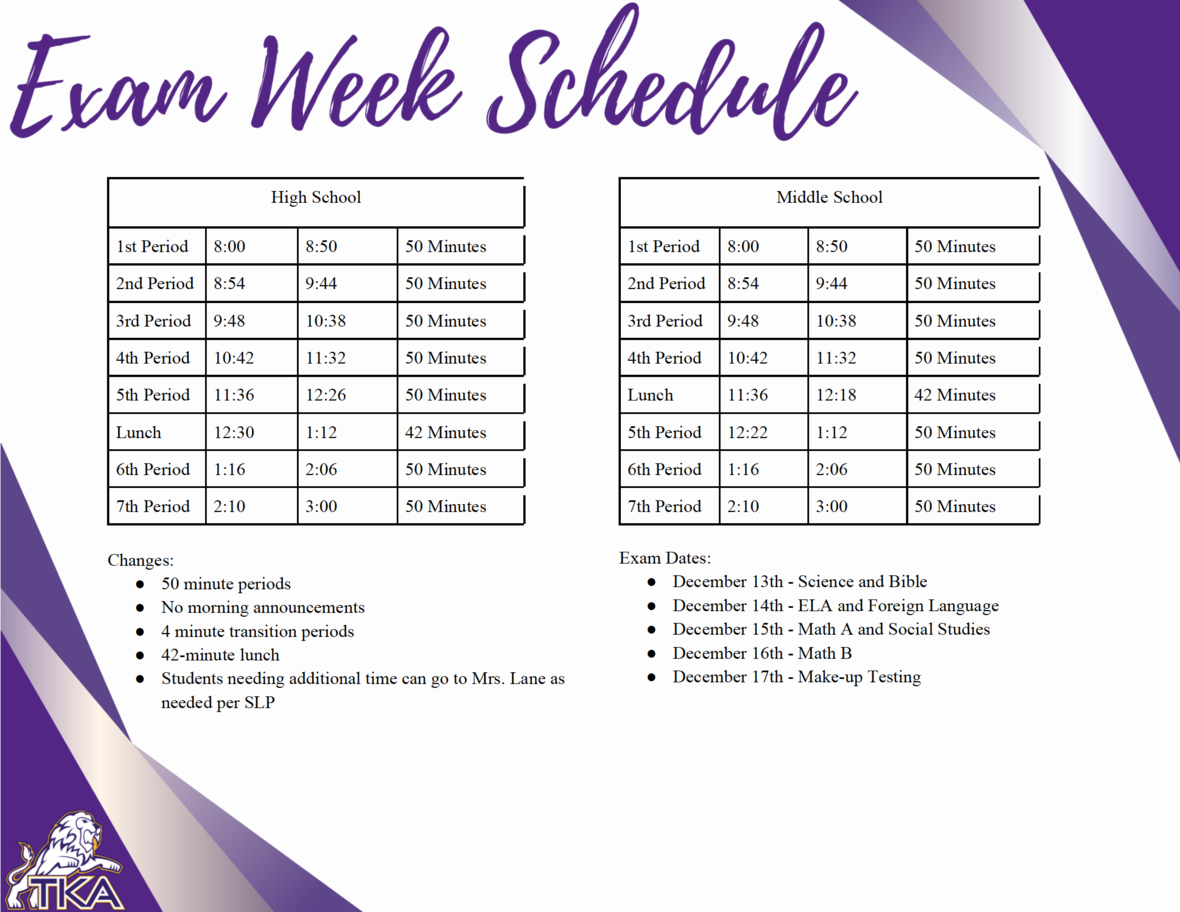table div table+table+table+table+table+table+table+table+table+table+table+table+table+table+table+table+table+table+table+table+table+table div table{width:100%;padding:0}table div table+table+table+table+table+table+table+table+table+table+table+table+table+table+table+table+table+table+table+table+table+table div table img{width:96.23%;padding:0;float:none}table div table+table+table+table+table+table+table+table+table+table+table+table+table+table+table+table+table+table+table+table+table+table div table td{width:100%;padding:0 1.88% 18px}/* styles */# Lost and Found

PreK and Elementary families, please be sure to check the Lost and Found in Ogle for any missing items prior to Christmas break. Any leftover items will be donated during the Christmas holidays.

 table div table+table+table+table+table+table+table+table+table+table+table+table+table+table+table+table+table+table+table+table+table+table+table+table div table{width:100%;padding:0}table div table+table+table+table+table+table+table+table+table+table+table+table+table+table+table+table+table+table+table+table+table+table+table+table div table img{width:96.23%;padding:0;float:none}table div table+table+table+table+table+table+table+table+table+table+table+table+table+table+table+table+table+table+table+table+table+table+table+table div table td{width:100%;padding:0 1.88% 18px}/* styles */# Upcoming Weekly Sporting Events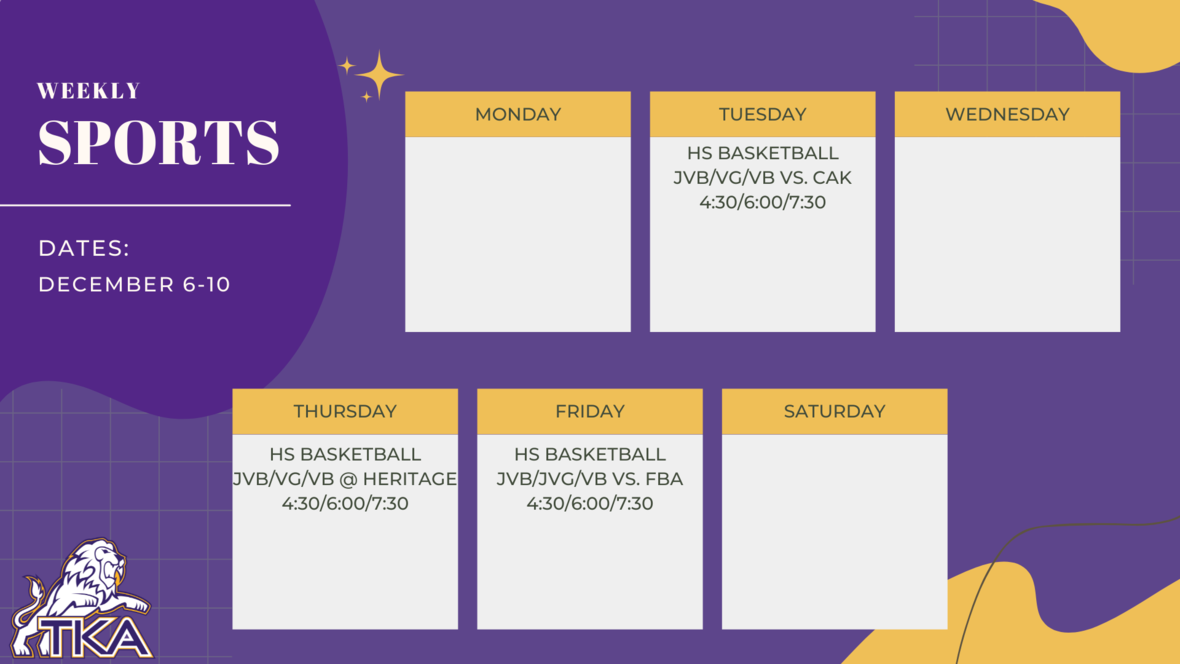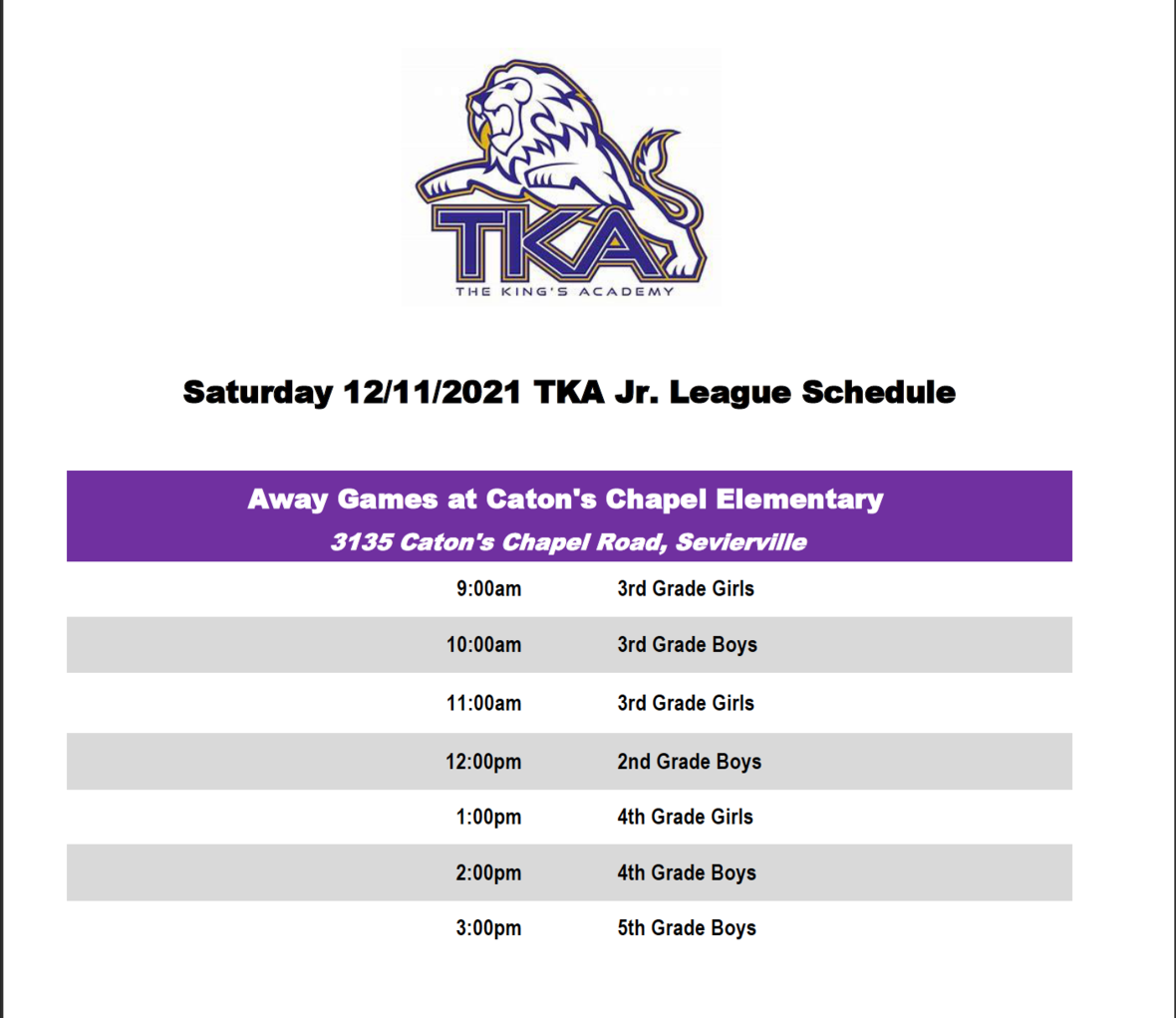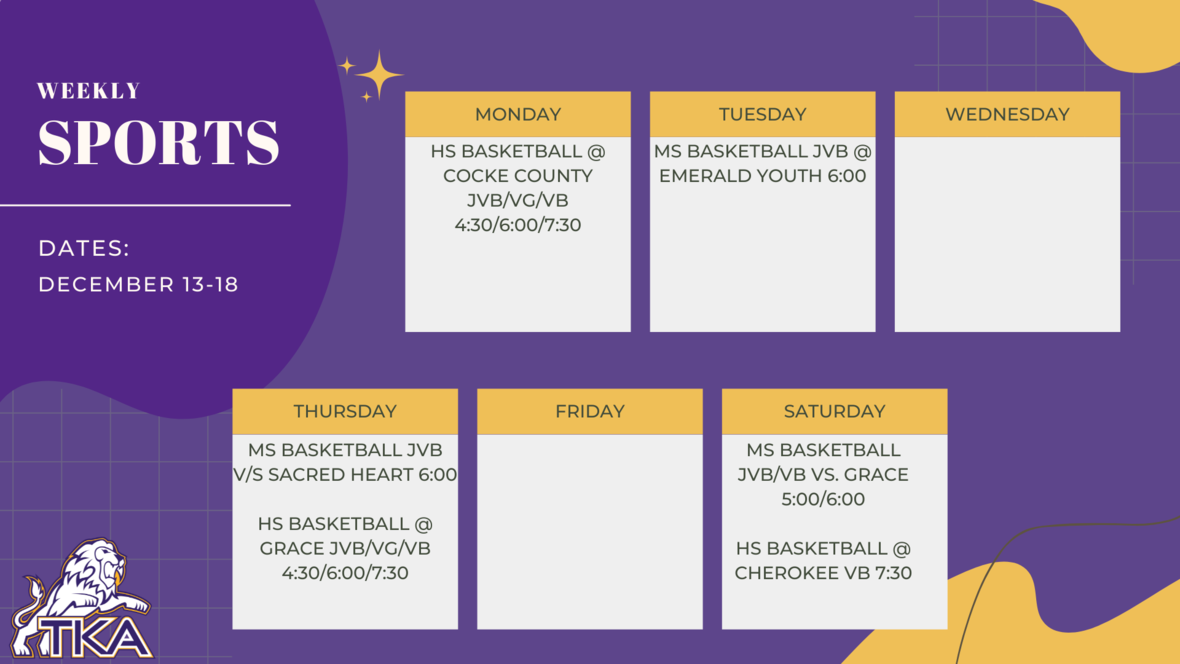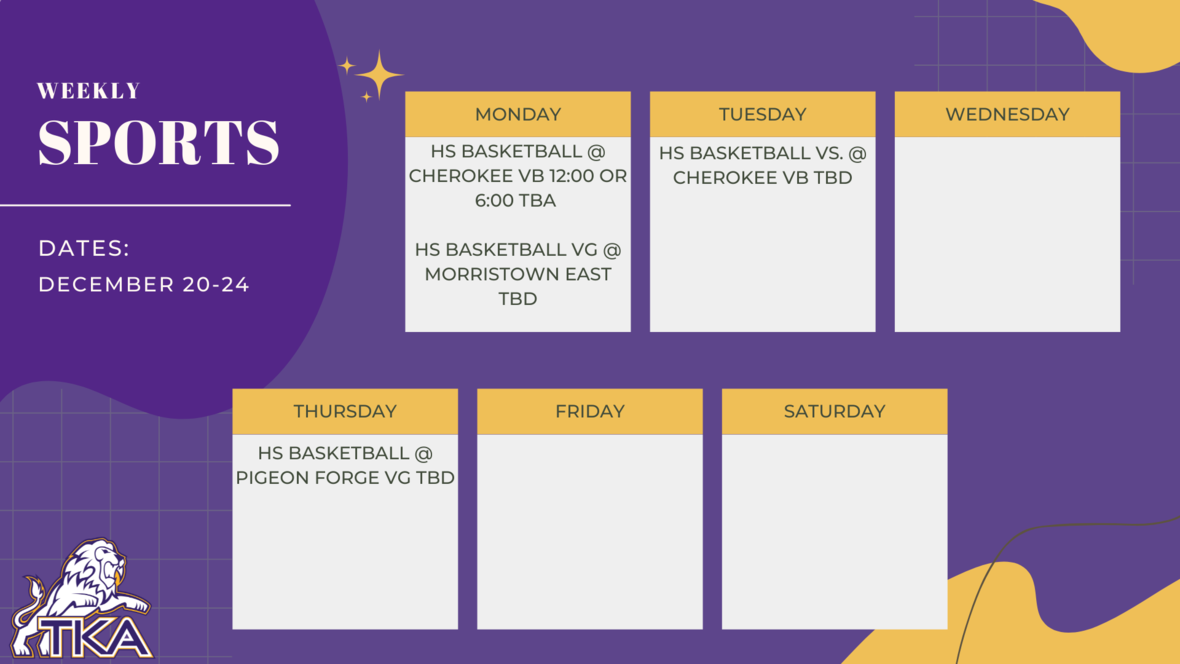table div table+table+table+table+table+table+table+table+table+table+table+table+table+table+table+table+table+table+table+table+table+table+table+table+table+table+table+table+table+table div table{width:100%;padding:0}table div table+table+table+table+table+table+table+table+table+table+table+table+table+table+table+table+table+table+table+table+table+table+table+table+table+table+table+table+table+table div table img{width:96.23%;padding:0;float:none}table div table+table+table+table+table+table+table+table+table+table+table+table+table+table+table+table+table+table+table+table+table+table+table+table+table+table+table+table+table+table div table td{width:100%;padding:0 1.88% 18px}/* styles */Winter Preview Night for prospective families will be held on Friday, January 21, 2022, at 6:00 in the Brandes Cafeteria. Please refer friends and family who may be interested in learning more about TKA to join us for a night of information, fellowship, and basketball! RSVP by January 17 by clicking here!

 table div table+table+table+table+table+table+table+table+table+table+table+table+table+table+table+table+table+table+table+table+table+table+table+table+table+table+table+table+table+table+table+table div table{width:100%;padding:0}table div table+table+table+table+table+table+table+table+table+table+table+table+table+table+table+table+table+table+table+table+table+table+table+table+table+table+table+table+table+table+table+table div table img{width:96.23%;padding:0;float:none}table div table+table+table+table+table+table+table+table+table+table+table+table+table+table+table+table+table+table+table+table+table+table+table+table+table+table+table+table+table+table+table+table div table td{width:100%;padding:0 1.88% 18px}/* styles */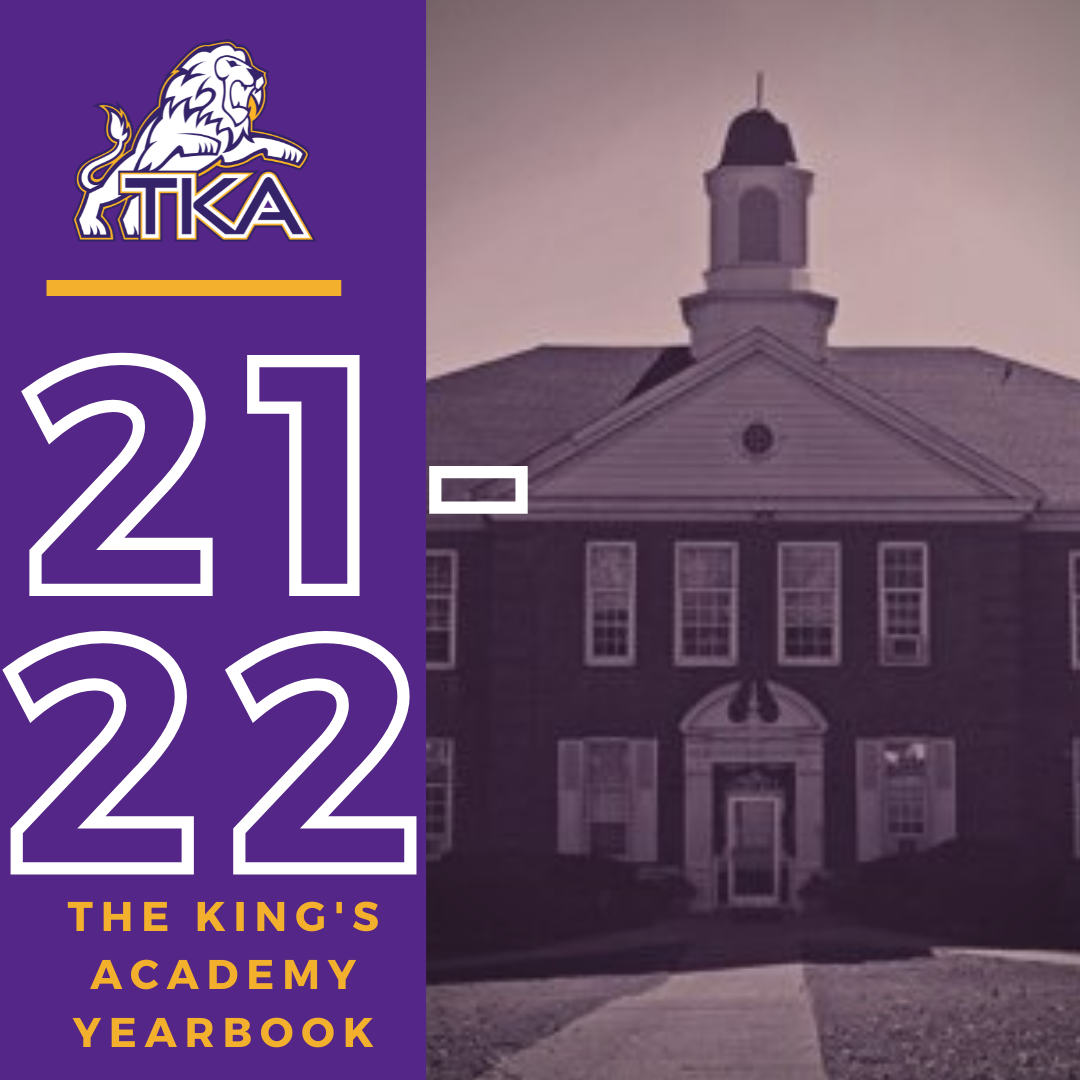# 2021-2022 Yearbook

The price for the 2021-2022 TKA yearbook is \$55. All grades, K3-12 will have the same yearbook. The slight price increase is because we are only going to have one larger all-school book.

All yearbook orders can be placed online--here is the link.

Senior Tributes and Business ads are available for purchase at the bottom of the link listed here.

For any questions regarding yearbooks, email Mr. Josh Smith at jsmith@thekingsacademy.net.

 table div table+table+table+table+table+table+table+table+table+table+table+table+table+table+table+table+table+table+table+table+table+table+table+table+table+table+table+table+table+table+table+table+table+table div table{width:100%;padding:0}table div table+table+table+table+table+table+table+table+table+table+table+table+table+table+table+table+table+table+table+table+table+table+table+table+table+table+table+table+table+table+table+table+table+table div table img{width:96.23%;padding:0;float:none}table div table+table+table+table+table+table+table+table+table+table+table+table+table+table+table+table+table+table+table+table+table+table+table+table+table+table+table+table+table+table+table+table+table+table div table td{width:100%;padding:0 1.88% 18px}/* styles */# TKA Scrip

As the holidays are approaching and Christmas shopping is beginning, don't forget about our TKA Scrip program!

Scrip is a no-selling program that allows families to save money on tuition and raise money for TKA by purchasing gift cards from over 975 businesses! Gift cards can be purchased in person each Friday morning from 7:45-8:15, or orders can be made online by clicking here. The enrollment code is: FC75F12F26641

Thank you for taking time to support our TKA Scrip program!

 table div table+table+table+table+table+table+table+table+table+table+table+table+table+table+table+table+table+table+table+table+table+table+table+table+table+table+table+table+table+table+table+table+table+table+table+table div table{width:100%;padding:0}table div table+table+table+table+table+table+table+table+table+table+table+table+table+table+table+table+table+table+table+table+table+table+table+table+table+table+table+table+table+table+table+table+table+table+table+table div table img{width:96.23%;padding:0;float:none}table div table+table+table+table+table+table+table+table+table+table+table+table+table+table+table+table+table+table+table+table+table+table+table+table+table+table+table+table+table+table+table+table+table+table+table+table div table td{width:100%;padding:0 1.88% 18px}/* styles */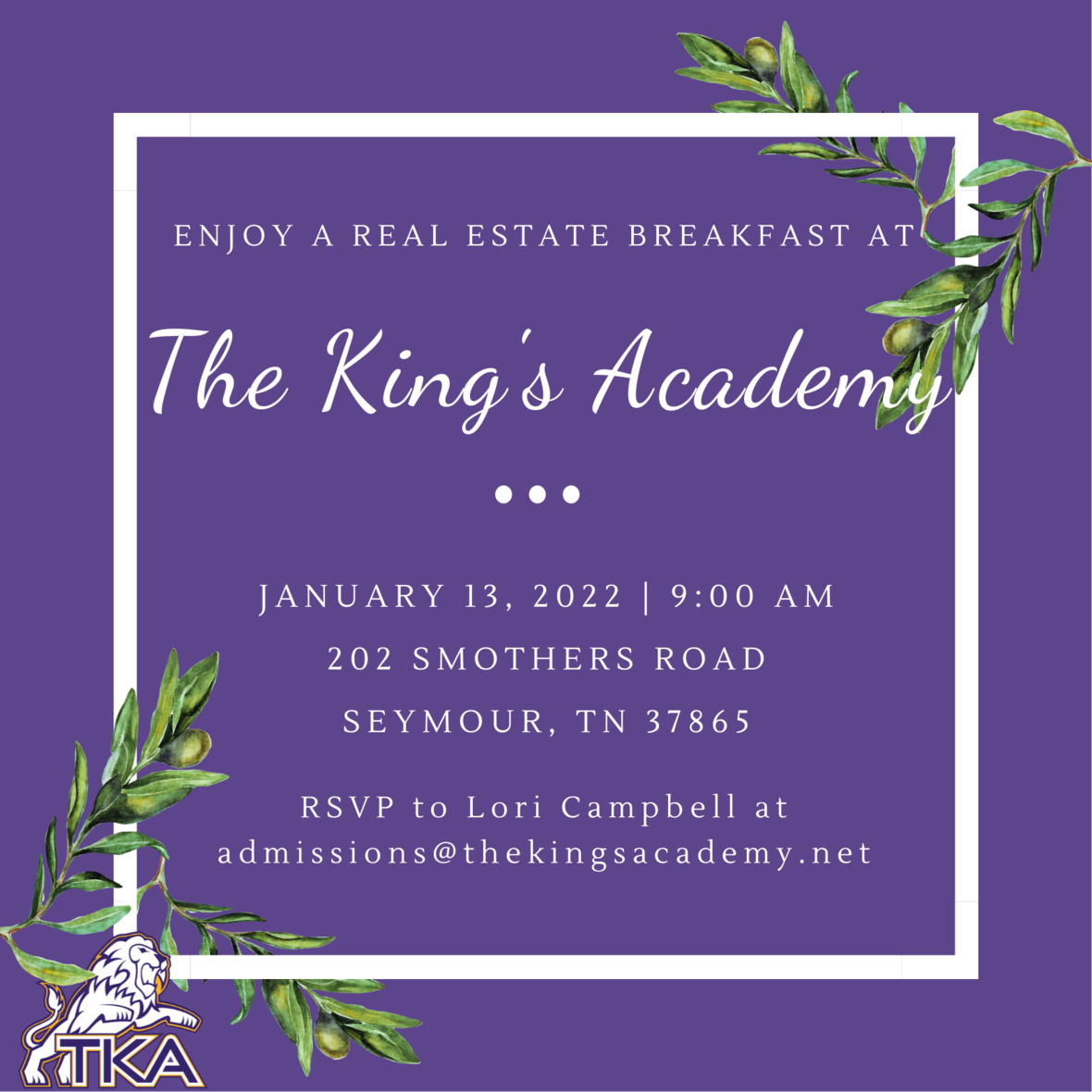# Real Estate Breakfast

Seymour and the surrounding area has been growing quickly, with many new prospective families moving into our community. In an effort to share the story of TKA with our neighbors, we would like to invite local realtors and real estate agents to breakfast on Thursday, January 13, at 9:00 in the Brandes Cafeteria. Please RSVP by January 5 to reserve your seat and informational materials. Click here to RSVP.

 table div table+table+table+table+table+table+table+table+table+table+table+table+table+table+table+table+table+table+table+table+table+table+table+table+table+table+table+table+table+table+table+table+table+table+table+table+table+table div table{width:100%;padding:0}table div table+table+table+table+table+table+table+table+table+table+table+table+table+table+table+table+table+table+table+table+table+table+table+table+table+table+table+table+table+table+table+table+table+table+table+table+table+table div table img{width:96.23%;padding:0;float:none}table div table+table+table+table+table+table+table+table+table+table+table+table+table+table+table+table+table+table+table+table+table+table+table+table+table+table+table+table+table+table+table+table+table+table+table+table+table+table div table td{width:100%;padding:0 1.88% 18px}/* styles */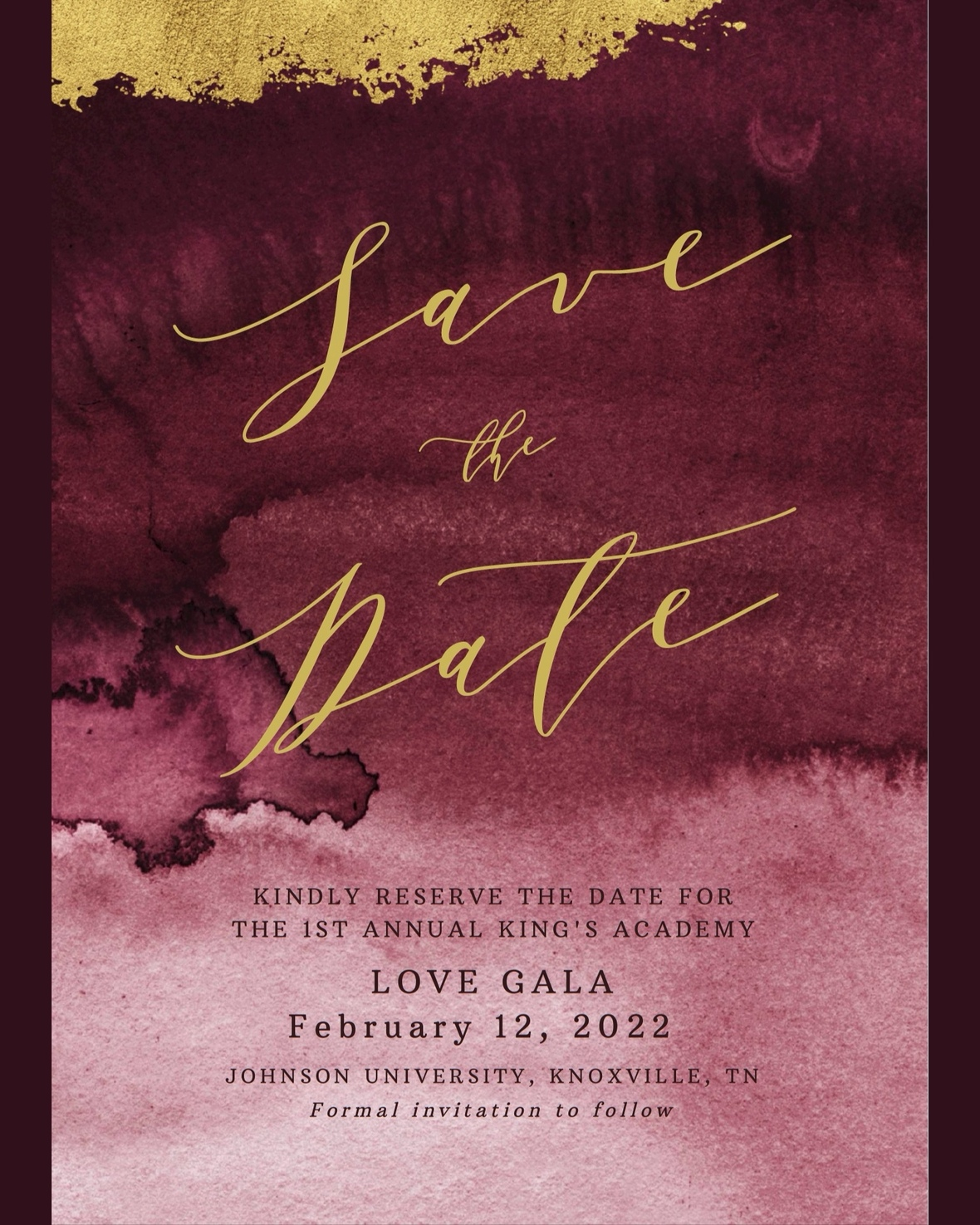# Love Gala

Save the date for the first annual Love Gala hosted by The King's Academy. The Love Gala will be on February 12, 2022, at Johnson University in Knoxville, TN. Plan on joining us for a night of fellowship, an exciting auction with great items, and a time to honor our alumni, teachers, and those who have left a legacy at TKA.

Each ticket purchased for the event will have an opportunity to win FREE tuition for one student for the 2022-23 academic year. Additional tuition remission opportunity tickets are available for purchase at the door for \$25 per opportunity. All opportunity drawing tickets are Non-Transferable. Only the student drawn is eligible for tuition remission. Must be present to receive tuition remission.

 table div table+table+table+table+table+table+table+table+table+table+table+table+table+table+table+table+table+table+table+table+table+table+table+table+table+table+table+table+table+table+table+table+table+table+table+table+table+table+table+table div table{width:100%;padding:0}table div table+table+table+table+table+table+table+table+table+table+table+table+table+table+table+table+table+table+table+table+table+table+table+table+table+table+table+table+table+table+table+table+table+table+table+table+table+table+table+table div table img{width:96.23%;padding:0;float:none}table div table+table+table+table+table+table+table+table+table+table+table+table+table+table+table+table+table+table+table+table+table+table+table+table+table+table+table+table+table+table+table+table+table+table+table+table+table+table+table+table div table td{width:100%;padding:0 1.88% 18px}/* styles *//* styles */
 table div table+table+table+table+table+table+table+table+table+table+table+table+table+table+table+table+table+table+table+table+table+table+table+table+table+table+table+table+table+table+table+table+table+table+table+table+table+table+table+table+table+table+table div table{width:100%;padding:0}table div table+table+table+table+table+table+table+table+table+table+table+table+table+table+table+table+table+table+table+table+table+table+table+table+table+table+table+table+table+table+table+table+table+table+table+table+table+table+table+table+table+table+table div table img{width:96.23%;padding:0;float:none}table div table+table+table+table+table+table+table+table+table+table+table+table+table+table+table+table+table+table+table+table+table+table+table+table+table+table+table+table+table+table+table+table+table+table+table+table+table+table+table+table+table+table+table div table td{width:100%;padding:0 1.88% 18px}/* styles */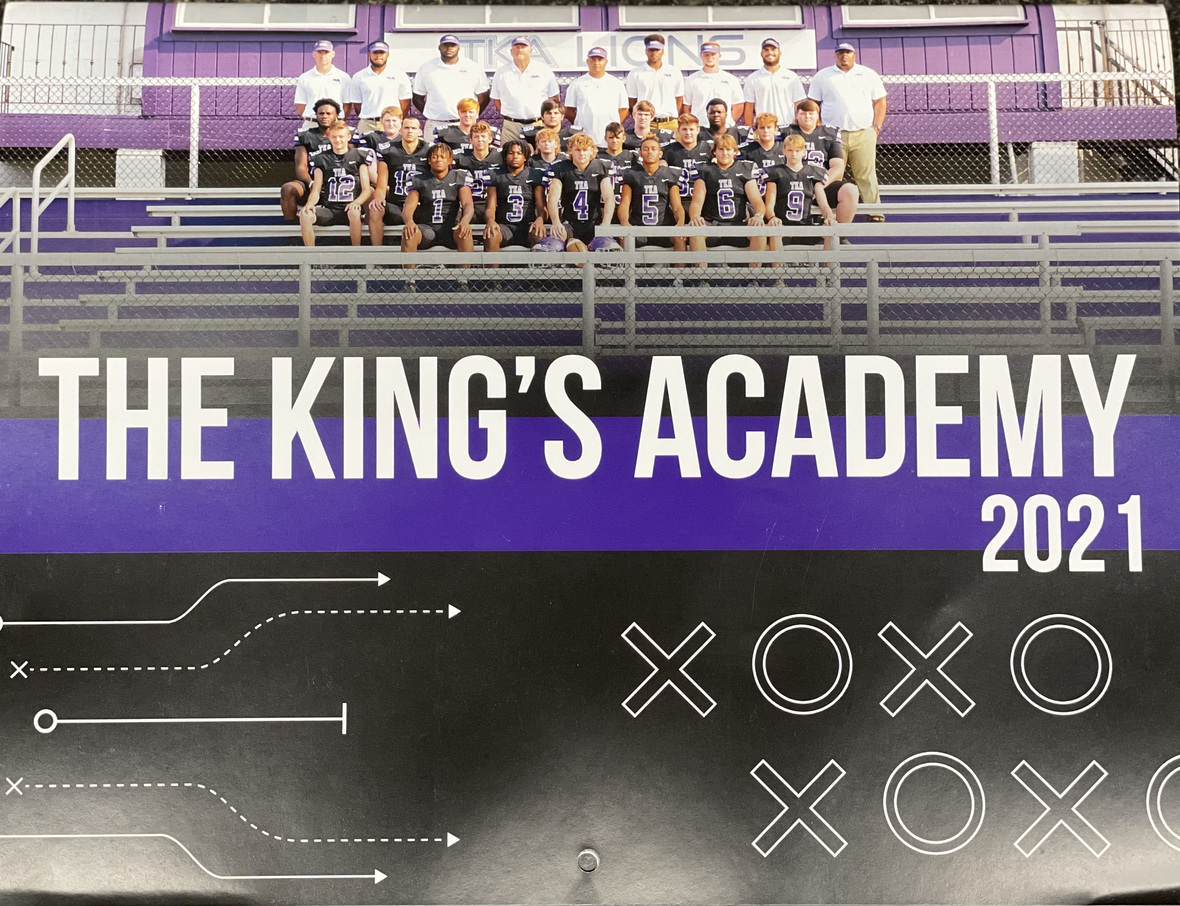# 2021-2022 Football Calendar

This year's football calendars are for sale for \$10! Stop by the school office to pick up your copy today!

 table div table+table+table+table+table+table+table+table+table+table+table+table+table+table+table+table+table+table+table+table+table+table+table+table+table+table+table+table+table+table+table+table+table+table+table+table+table+table+table+table+table+table+table+table+table div table{width:100%;padding:0}table div table+table+table+table+table+table+table+table+table+table+table+table+table+table+table+table+table+table+table+table+table+table+table+table+table+table+table+table+table+table+table+table+table+table+table+table+table+table+table+table+table+table+table+table+table div table img{width:96.23%;padding:0;float:none}table div table+table+table+table+table+table+table+table+table+table+table+table+table+table+table+table+table+table+table+table+table+table+table+table+table+table+table+table+table+table+table+table+table+table+table+table+table+table+table+table+table+table+table+table+table div table td{width:100%;padding:0 1.88% 18px}/* styles */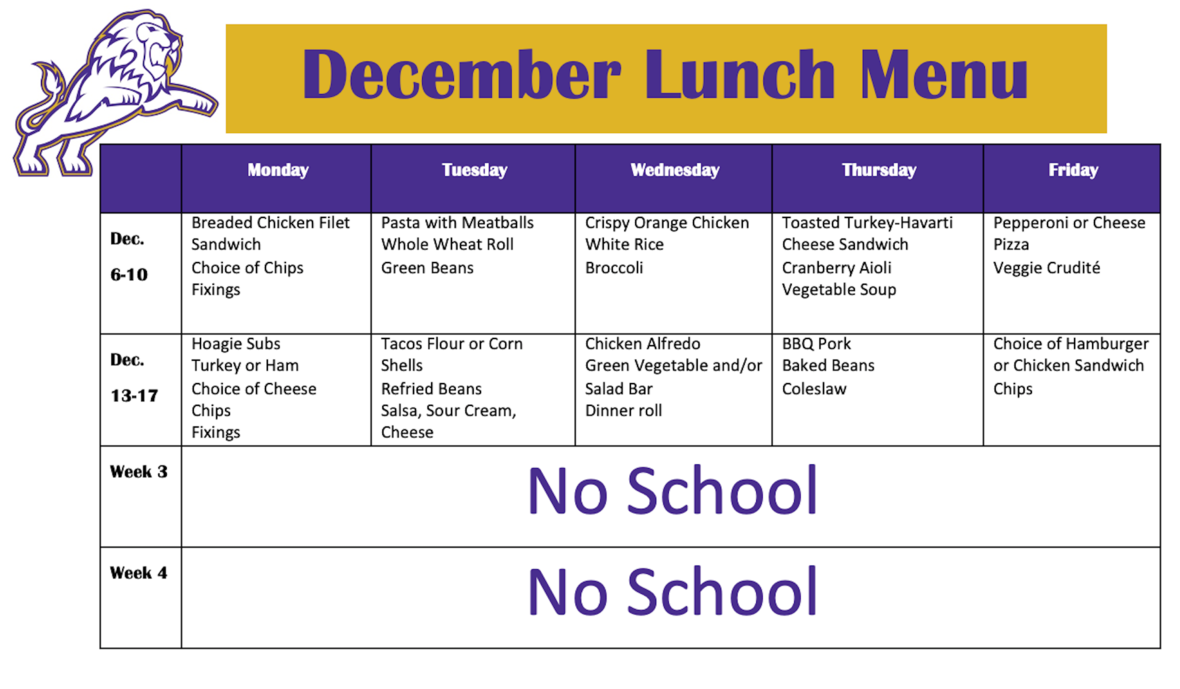table div table+table+table+table+table+table+table+table+table+table+table+table+table+table+table+table+table+table+table+table+table+table+table+table+table+table+table+table+table+table+table+table+table+table+table+table+table+table+table+table+table+table+table+table+table+table+table div table{width:100%;padding:0}table div table+table+table+table+table+table+table+table+table+table+table+table+table+table+table+table+table+table+table+table+table+table+table+table+table+table+table+table+table+table+table+table+table+table+table+table+table+table+table+table+table+table+table+table+table+table+table div table img{width:96.23%;padding:0;float:none}table div table+table+table+table+table+table+table+table+table+table+table+table+table+table+table+table+table+table+table+table+table+table+table+table+table+table+table+table+table+table+table+table+table+table+table+table+table+table+table+table+table+table+table+table+table+table+table div table td{width:100%;padding:0 1.88% 18px}/* styles */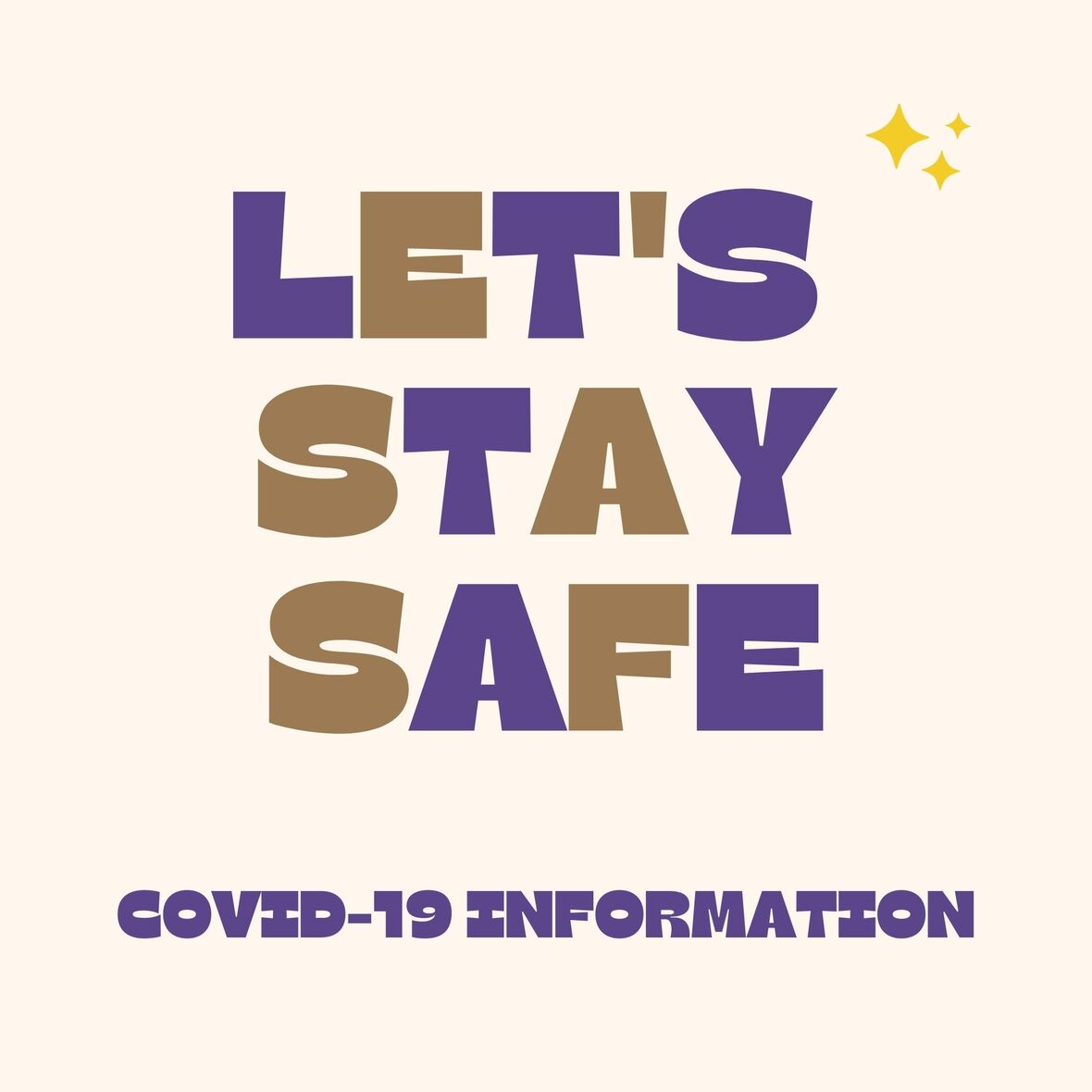# Covid-19 Procedures

While we are excited to offer in-person education, we must maintain a safe learning environment for all students, faculty/staff, and additional stakeholders.

To do so, we will be working closely with the Sevier County Health Department regarding Covid-19 protocols.

 table div table+table+table+table+table+table+table+table+table+table+table+table+table+table+table+table+table+table+table+table+table+table+table+table+table+table+table+table+table+table+table+table+table+table+table+table+table+table+table+table+table+table+table+table+table+table+table+table+table div table{width:100%;padding:0}table div table+table+table+table+table+table+table+table+table+table+table+table+table+table+table+table+table+table+table+table+table+table+table+table+table+table+table+table+table+table+table+table+table+table+table+table+table+table+table+table+table+table+table+table+table+table+table+table+table div table img{width:96.23%;padding:0;float:none}table div table+table+table+table+table+table+table+table+table+table+table+table+table+table+table+table+table+table+table+table+table+table+table+table+table+table+table+table+table+table+table+table+table+table+table+table+table+table+table+table+table+table+table+table+table+table+table+table+table div table td{width:100%;padding:0 1.88% 18px}/* styles */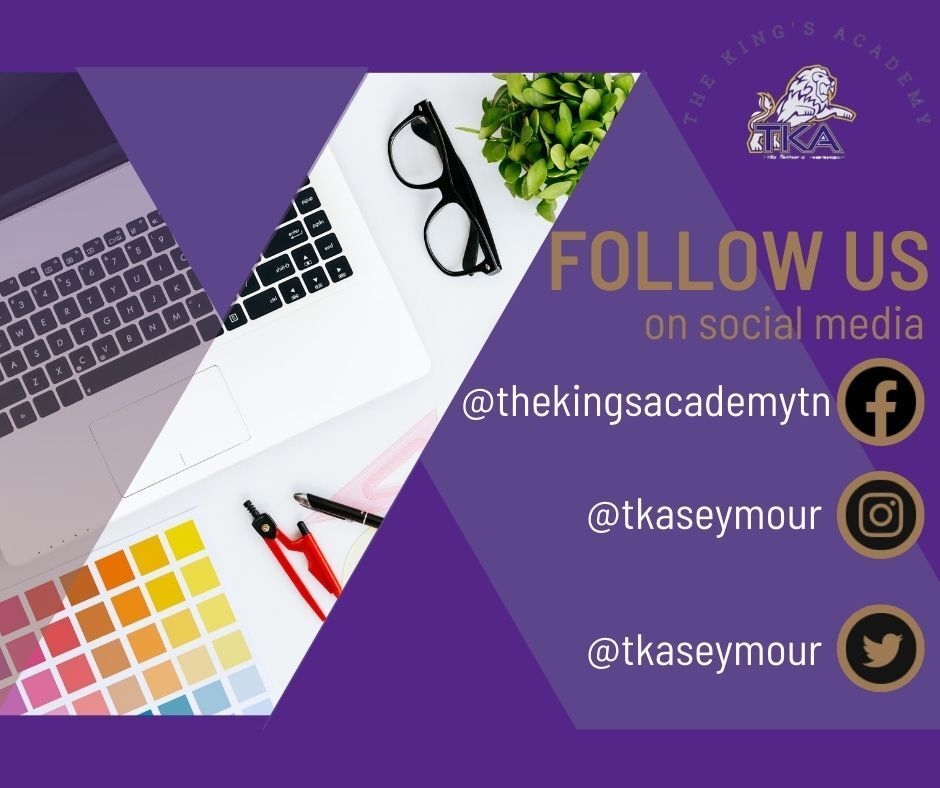table div table+table+table+table+table+table+table+table+table+table+table+table+table+table+table+table+table+table+table+table+table+table+table+table+table+table+table+table+table+table+table+table+table+table+table+table+table+table+table+table+table+table+table+table+table+table+table+table+table+table+table div table{width:100%;padding:0}table div table+table+table+table+table+table+table+table+table+table+table+table+table+table+table+table+table+table+table+table+table+table+table+table+table+table+table+table+table+table+table+table+table+table+table+table+table+table+table+table+table+table+table+table+table+table+table+table+table+table+table div table img{width:96.23%;padding:0;float:none}table div table+table+table+table+table+table+table+table+table+table+table+table+table+table+table+table+table+table+table+table+table+table+table+table+table+table+table+table+table+table+table+table+table+table+table+table+table+table+table+table+table+table+table+table+table+table+table+table+table+table+table div table td{width:100%;padding:0 1.88% 18px}/* styles */